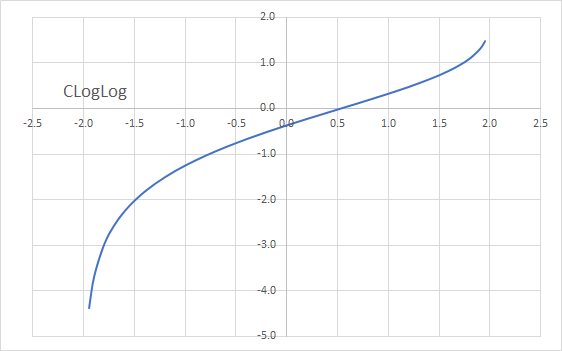# CLOGLOG - Complementary Log-Log Transform

Computes the complementary log-log transformation, including its inverse.

## Syntax

CLOGLOG(X, Lo, Hi, Return)

X
is the real value(s) for which we compute the transformation: a single value or a one-dimensional array of cells (e.g., rows or columns)).
Lo
is the x-domain lower limit. If missing, Lo is assumed to be 0.
Hi
is the x-domain upper limit. If missing, Hi is assumed to be 1.
Return
is a number that determines the type of return value: 1 (or missing) = C-Log-Log, 2 = Inverse C-Log-Log.
Return Description
1 or omitted C-Log-Log Transform.
2 The inverse of the C-Log-Log Transform.

## Remarks

1. The X value(s) must be between Lo and Hi (exclusive).
2. The complementary log-log link function is commonly used for parameters that lie in the unit interval.
3. The standard complementary log-log transformation is defined as follows:
$$y=\textit{CLogLog}(x)=ln{(-ln{(1-x)})}$$ And,
$$x=\textit{CLogLog}^{-1}(y)=1-e^{-e^y}$$
Where:
• $x_{t}$ is the input value of the input time series at time $t$. X must be between 0 and 1, exclusive.
• $y_{t}$ is the transformed complementary log-log value at time $t$.
• $\textit{CLogLog}^{-1}(y)$ is the inverse complementary log-log function.
4. To support a generic interval (a, b), we perform the following mapping:
$$z=\frac{x - a}{b - a}$$ So, the revised transform function is expressed as follows:
$$y=\ln{(-\ln{(\frac{b-x}{b-a})})}$$ The inverse transform is expressed as follows:
$$-e^y=\ln{(\frac{b-x}{b-a})}\Rightarrow x=b-(b-a)e^{-e^y}$$
5. The transform first-order derivative is calculated as follows:
$$\frac{dy}{dx}=\frac{-1}{(b-a)\ln{\frac{b-x}{b-a}}}$$
6. The transform first-order derivative (i.e., $\frac{dy}{dx}$ is positive for all x-values and goes to infinity as $x$ approaches the interval endpoints.
7. In essence, the complementary log-log function converts a bounded x-range from (Lo, Hi) to $(-\infty, \infty)$.8. Unlike Logit and Probit, the complementary log-log transform is asymmetrical.